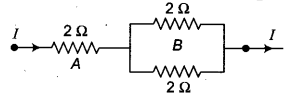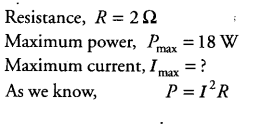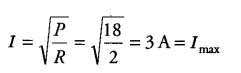# Three ,20 resistors, A, B and C are connected as shown in figure

Three ,20 resistors, A, B and C are connected as shown in figure. Each of them dissipates energy and can with stand a maximum power of 18 W without melting. Find the maximum current that can flow through the three resistors.Maximum current that can flow through 20 resistor is 3 A. This current divides along B and C because in parallel combination, voltage across B and C remain same and hence, I\;\alpha \;\frac{1}{R} . Since, B and C have same resistance 2 \Omega each, therefore, same current i.e. \frac{3}{2} = 1.5
A flows through B and C.